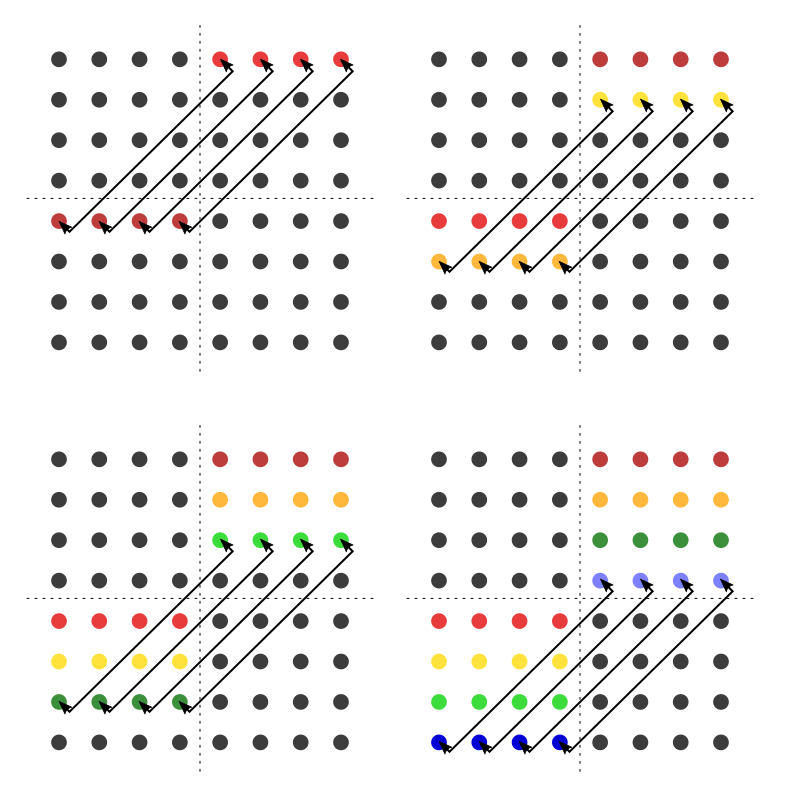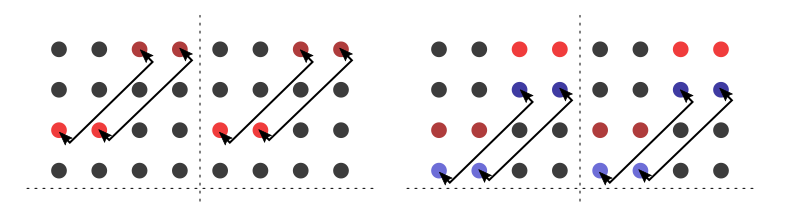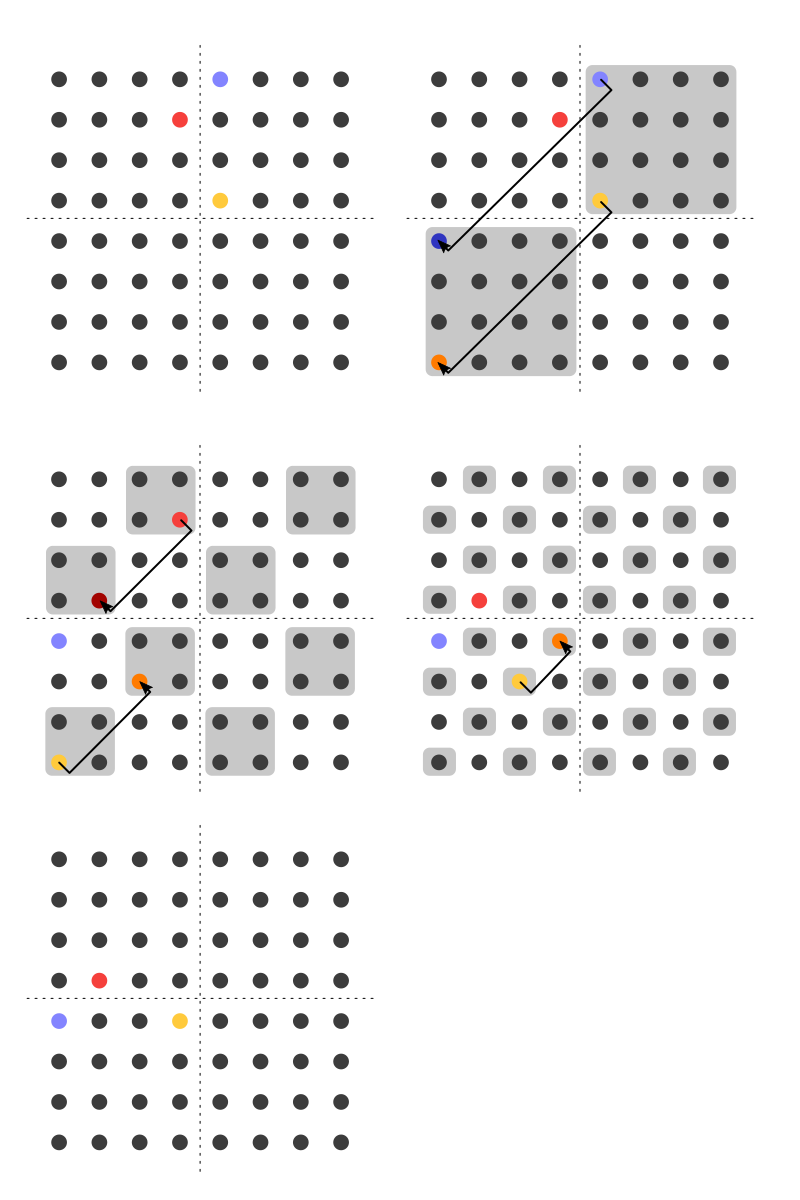# Transposing bits

✍️ → Written on 2021-07-23 in 3273 words. Part of cs IT-security pqcrypto software-development

## Motivation

Consider 64 integers of 64-bit precision as a matrix with (64×64=) 4096 elements. How does one compute the transpose of this matrix?

Classic McEliece is one of the finalists of the NIST PQC standardization process. The reference implementation contains the transpose.c file which features an efficient transpose function. We will look into this function in detail in this article.

## Fundamentals

### Transpose function

Let M be a matrix. Let M[r] denote the r-th row of M and M[r][c] denote the j-th element of the i-th row of M. Then a transpose operation swaps elements M[r][c] and M[c][r].

From a visual point of view, consider the matrix on the left (entries are 0 or 1). It will be transposed into the matrix on the right:

``````1 0 1 0  ⇒  1 1 1 1
1 0 1 1  ⇒  0 0 0 1
1 0 0 0  ⇒  1 1 0 1
1 1 1 1  ⇒  0 1 0 1``````

You can observe the diagonal from top-left to bottom-right is preserved. Other elements are mirrored along this axis. So the top-right element is put into the bottom-left corner.

### Bitwise operations revisited

This is fundamental to every system-level programmer, but I will revise how bitwise operations work first. I will use hexadecimal notation here (and thus nibbles), because binary notation is a non-standard in the C programming language.

``````uint16_t a = 0xAABB;
uint16_t b = (a & 0xFF00);  // gives 0xAA00
uint16_t c = (a & 0x00FF);  // gives 0x00BB
uint16_t d = (a & 0xF0F0);  // gives 0xA0B0``````

We can select individual bits (or here: always groups of 4 bits) by applying the bitwise AND operation with a bitmask. Bitmasks have ones at positions where the value shall be extracted and zeros at positions where the value shall be neglected.

``````uint16_t a = 0xAABB;
uint16_t b = a >> 4;             // gives 0x0AAB
uint16_t c = a >> 8;             // gives 0x00AA
uint16_t d = (a & 0xFF00) >> 4;  // gives 0x0AA0``````

Shifting right by N positions discards N bits on the right and introduces N zero bits on the left. We can also apply bitwise operations to combine bits of several words.

``````uint16_t x = 0xAABB;
uint16_t y = 0xCCDD;

uint16_t a = (x & 0xFF00) | (y & 0x00FF);        // gives 0xAADD
uint16_t b = (x & 0xF0F0) | (y & 0x0F0F);        // gives 0xACBD
uint16_t c = (x & 0xFF00) | ((y & 0xFF00) >> 8); // gives 0xAACC``````

The OR operation returns a one bit, if either the left or right argument has a one bit. If the bits are set mutually exclusive, it feels like OR “concatenates” the bits.

## Implementation

Now, we can consider implementations of the transpose function. First, we will just swap individual elements in the matrix. However, we will recognize that we can implement it more efficiently.

### A naïve approach

Looking at the definition of transpose above, we have to “swap elements M[r][c] and M[c][r]”. So fundamentally, we can simply implement it using the following routine:

``````for (r = 0; r < 64; r++)
for (c = r + 1; c < 64; c++)
swap(in[r][c], in[c][r]);``````

We iterate over half of the matrix elements and swap them with their respective counterpart. Be aware that iterating the entire matrix would apply each swap operation twice and we would end up with the original array.

But there are two open questions: What is the data type of matrix in? How does function swap work?

They are actually related. The answers are that in is an array of 64 integers of 64-bit precision. So we cannot use `in[r][c]` to index a bit. And swap actually needs to be implemented with bitwise operations. Using bitwise operation, we can pick one bit, the other bit and assign them at their swapped position:

``````uint64_t all_ones = 0xFFFFFFFFFFFFFFFF;
ssize_t r, c;
for (r = 0; r < 64; r++)
for (c = r + 1; c < 64; c++) {
uint64_t bit1 = (in[r] & ((uint64_t)1 << c)) >> c;
uint64_t bit2 = (in[c] & ((uint64_t)1 << r)) >> r;

in[r] = (in[r] & ((uint64_t)all_ones ^ ((uint64_t)1 << c))) | ((uint64_t)bit2 << c);
in[c] = (in[c] & ((uint64_t)all_ones ^ ((uint64_t)1 << r))) | ((uint64_t)bit1 << r);
}``````

This is a complete implementation of the transpose function. But what is its runtime? We iterate over half of the bits of the matrix and make two integer assignments there. Thus if n is the number of words and we want to model the number of assignments, we have 2・(n²-n)/2 = n² - n = Ο(n²).

### Next approach: Considering a base case

Let us observe a base case.

``````1 0  ⇒  1 1        a b  ⇒  a c
1 0  ⇒  0 0        c d  ⇒  b d``````

Consider the 2×2 matrix on the left. Here, we keep the elements top-left and bottom-right in place. However, the elements top-right and bottom-left swap places.

Let {a, b, c, d} be four bits. Then we get the structure on the right. Can this structure be generalized? Can {a, b, c, d} be themselves matrices?

### Thinking in submatrices

The idea is pretty simple:

1. We split the matrix into 4 submatrices of uniform size.

2. We swap the top-right and bottom-left submatrices.

3. We apply the idea recursively to each submatrix.

To illustrate this process, we first look at the entire matrix (step 1). We use an 8×8 matrix here due to layout restrictions. This implies that our submatrices have a size of 4×4 respectively (step 2). Since each access happens on a word/row level, we still need 4 operations to actually swap the two submatrices. This step is illustrated in the following image:Now we repeatedly apply the process to the submatrices (step 3). Now our matrix has size has size 4×4 and submatrices have size 2×2.

But here, an optimization comes into play: Since entire words are loaded anyways, we can actually process 2 submatrices at once. We apply the two swaps simultaneously for the two top submatrices:Then we continue with the bottom two submatrices. Then we recurse again. We stop once we have swapped matrices of size 1×1.

### Correctness

Does it actually transpose all the desired values? Yes.

• The base case shows that individual elements are put into place.

• Multiple elements are not put in their correct place. For example, elements close to the diagonal will be put far away from the diagonal.

• But they are put into place if those elements become transposed themselves. And this is what we do by recursing.

Not convinced yet? Maybe tracking individual elements helps one to see this. In this illustration, I use a gray background to highlight considered submatrices:### Runtime

• We have a recursion depth of log(n).

• We need to access each element in a submatrix. But we do this simultaneously for several submatrices. Since, we care only about word assignments, we can simple consider that we write all words at each recursion level. Thus we get n assignments.

In this algorithm, we get Ο(n・log(n)) integer assignments.

Let us finish the algorithm by implementing it in C.

### The optimized implementation

You can look at the reference implementation contained in the round 3 submissions. A more direct link is Tung Chou‘s paper “McBits Revisited”. In this paper, Figure 2 (page 8) shows source code similar to this (I added the variable declarations here):

``````const uint64_t mask = {
{0X5555555555555555, 0XAAAAAAAAAAAAAAAA},
{0X3333333333333333, 0XCCCCCCCCCCCCCCCC},
{0X0F0F0F0F0F0F0F0F, 0XF0F0F0F0F0F0F0F0},
{0X00FF00FF00FF00FF, 0XFF00FF00FF00FF00},
{0X0000FFFF0000FFFF, 0XFFFF0000FFFF0000},
{0X00000000FFFFFFFF, 0XFFFFFFFF00000000}
};

int i, j, p, s;
uint64_t idx0, idx1, x, y;

for (j = 5; j >= 0; j--)
{
s = 1 << j;
for (p = 0; p < 32/s; p++)
for (i = 0; i < s; i++)
{
idx0 = p*2*s + i;
idx1 = p*2*s + i + s;
in[idx0] = x;
in[idx1] = y;
}
}``````
• `mask` is a bitmask to extract all bits of submatrices of one word. Since we need to swap the top-right submatrix and bottom-left submatrix, we need two selector bitmasks. I tend to call them high bits and low bits (following the notions of MSB/LSB).

• `j` iterates over each submatrix selection.

• `s` denotes the width of a submatrix.

• Loops over `p` and `i` provide us indices for the words, we want to swap bits in. `p` defines how many submatrices occur per column in the 64×64 matrix. `i` is an iterator within each submatrix.

## Conclusion

This is a wonderful small optimization to compute transposes quicker. Its limitation is the word size of the computer (mostly 64 bits, but up to 512 bits). You can only compute the transpose more efficiently for binary matrices of these sizes. Thus, its usecases are limited. But as can be seen in the case of Classic McEliece it can be a core routine to speed up your cryptographic operations.

The algorithm occurs in “The Art of Computer Programming” Volume 4 Fascicle 1 on page 56 as exercise 54. The algorithm is provided as solution on page 158. Don Knuth attributes the exercise to R. W. Gosper (1985).

## Appendix: complete naïve implementation

``````#include <stdio.h>
#include <stdint.h>

void print_matrix(uint64_t m) {
int i, j;
for (i = 0; i < 64; i++) {
for (j = 0; j < 64; j++)
printf("%lu", (m[i] >> j) & 1);
printf("\n");
}
}

void transpose(uint64_t in) {
uint64_t all_ones = 0xFFFFFFFFFFFFFFFF;
ssize_t r, c;
for (r = 0; r < 64; r++)
for (c = r + 1; c < 64; c++) {
uint64_t bit1 = (in[r] & ((uint64_t)1 << c)) >> c;
uint64_t bit2 = (in[c] & ((uint64_t)1 << r)) >> r;

in[r] = (in[r] & ((uint64_t)all_ones ^ ((uint64_t)1 << c))) | ((uint64_t)bit2 << c);
in[c] = (in[c] & ((uint64_t)all_ones ^ ((uint64_t)1 << r))) | ((uint64_t)bit1 << r);
}
}

int main() {
int i;
uint64_t in;
for (i = 0; i < 32; i++) {
in[2*i] = 0xFFFF0000FFFF0000;
in[2*i+1] = 0x0000FFFF0000FFFF;
}
in |= 0x000000A000000000; // observation needle - where does this value end up?

printf("before:\n");
print_matrix(in);
transpose(in);
printf("after:\n");
print_matrix(in);
}``````

## Appendix: complete optimized implementation

``````#include <stdio.h>
#include <stdint.h>

{0X5555555555555555, 0XAAAAAAAAAAAAAAAA},
{0X3333333333333333, 0XCCCCCCCCCCCCCCCC},
{0X0F0F0F0F0F0F0F0F, 0XF0F0F0F0F0F0F0F0},
{0X00FF00FF00FF00FF, 0XFF00FF00FF00FF00},
{0X0000FFFF0000FFFF, 0XFFFF0000FFFF0000},
{0X00000000FFFFFFFF, 0XFFFFFFFF00000000}
};

void print_matrix(uint64_t m) {
int i, j;
for (i = 0; i < 64; i++) {
for (j = 0; j < 64; j++)
printf("%lu", (m[i] >> j) & 1);
printf("\n");
}
}

int main() {
int i, j, p, s;
uint64_t idx0, idx1, x, y;

// initialize `in`
uint64_t in;
for (i = 0; i < 32; i++) {
in[2*i] = 0xFFFF0000FFFF0000;
in[2*i+1] = 0x0000FFFF0000FFFF;
}
in |= 0x000000A000000000; // observation needle - where does this value end up?

// print before
printf("before:\n");
print_matrix(in);
printf("\n");

// apply Beneš network
for (j = 5; j >= 0; j--)
{
s = 1 << j;
for (p = 0; p < 32/s; p++)
for (i = 0; i < s; i++)
{
idx0 = p*2*s + i;
idx1 = p*2*s + i + s;
printf("apply iteration to indices %02lx and %02lx\n", idx0, idx1);